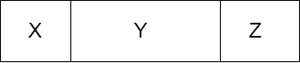Open In App

# Minimum number of deletions from front and back of given Array to make count of 0 and 1 equal

Given an array arr[] consisting of only 0 and 1. The task is to find the minimum number of deletions from the front and back of the array, such that the new modified array consists of an equal number of 0’s and 1’s.

Examples:

Input: arr[] = {1, 1, 0, 1}
Output: 2
Explanation: Two ones from the starting or first and last element can be removed

Input: arr[] = {0, 1, 1, 1, 0}
Output: 3
Explanation: First three elements can be removed or last three elements

Approach: The problem can be solved using greedy approach. As each element of the array can be either 0 or 1, so consider 0 as -1 and 1 as 1 itself. Then find the largest subarray that consists of an equal number of 1s and 0s. Then subtract the size of this subarray from the total size of the array. This approach works because at any moment the approach tries to keep the size of the subarray as large as possible so that only a minimum number of elements from the ends can be removed. See the following diagram for a better understanding.As it can be seen from the diagram, as the size of middle part y increases which consists of an equal number of 0s and 1s, automatically the size of part (x + z) decreases.

Below is the implementation of the above approach.

## C++

 `// C++ code for the above approach``#include ``using` `namespace` `std;` `// Function to calculate``// the minimum number of deletions``int` `solve(vector<``int``> arr)``{` `    ``// Size of the array``    ``int` `sz = arr.size();` `    ``// Store running sum of array``    ``int` `summe = 0;` `    ``// To store index of the sum``    ``unordered_map<``int``, ``int``> index;` `    ``// Initially sum is 0 with index -1``    ``index[summe] = -1;` `    ``// Store length of largest subarray``    ``int` `ans = 0, curr_len = 0;` `    ``for` `(``int` `i = 0; i < sz; i++) {` `        ``// If curr_element is 0, add -1``        ``if` `(arr[i] == 0)``            ``summe -= 1;` `        ``// If curr_element is 1, add 1``        ``else``            ``summe += 1;` `        ``// Check if sum already occurred``        ``if` `(index.find(summe) != index.end()) {` `            ``// Calculate curr subarray length``            ``curr_len = i - index[summe];` `            ``// Store the maximum value``            ``// in the ans``            ``ans = max(ans, curr_len);``        ``}` `        ``// Sum occurring for the first time``        ``// So store this sum with current index``        ``else``            ``index[summe] = i;``    ``}` `    ``// Return diff between size``    ``// and largest subarray``    ``return` `(sz - ans);``}` `// Driver code``int` `main()``{``    ``vector<``int``> arr = { 1, 1, 0, 1 };``    ``int` `val = solve(arr);``    ``cout << val;``}` `// This code is contributed by Samim Hossain Mondal.`

## Java

 `// Java code for the above approach``import` `java.util.*;` `class` `GFG{` `  ``// Function to calculate``  ``// the minimum number of deletions``  ``static` `int` `solve(``int``[] arr)``  ``{` `    ``// Size of the array``    ``int` `sz = arr.length;` `    ``// Store running sum of array``    ``int` `summe = ``0``;` `    ``// To store index of the sum``    ``HashMap index = ``new` `HashMap();` `    ``// Initially sum is 0 with index -1``    ``index.put(summe, -``1``);` `    ``// Store length of largest subarray``    ``int` `ans = ``0``, curr_len = ``0``;` `    ``for` `(``int` `i = ``0``; i < sz; i++) {` `      ``// If curr_element is 0, add -1``      ``if` `(arr[i] == ``0``)``        ``summe -= ``1``;` `      ``// If curr_element is 1, add 1``      ``else``        ``summe += ``1``;` `      ``// Check if sum already occurred``      ``if` `(index.containsKey(summe) ) {` `        ``// Calculate curr subarray length``        ``curr_len = i - index.get(summe);` `        ``// Store the maximum value``        ``// in the ans``        ``ans = Math.max(ans, curr_len);``      ``}` `      ``// Sum occurring for the first time``      ``// So store this sum with current index``      ``else``        ``index.put(summe, i);``    ``}` `    ``// Return diff between size``    ``// and largest subarray``    ``return` `(sz - ans);``  ``}` `  ``// Driver code``  ``public` `static` `void` `main(String[] args)``  ``{``    ``int` `[]arr = { ``1``, ``1``, ``0``, ``1` `};``    ``int` `val = solve(arr);``    ``System.out.print(val);``  ``}``}` `// This code is contributed by 29AjayKumar`

## Python3

 `# Python code to implement the given approach``from` `collections ``import` `defaultdict` `class` `Solution:``    ` `    ``# Function to calculate``    ``# the minimum number of deletions``    ``def` `solve(``self``, arr):` `        ``# Size of the array``        ``size ``=` `len``(arr)` `        ``# Store running sum of array``        ``summe ``=` `0` `        ``# To store index of the sum``        ``index ``=` `defaultdict(``int``)` `        ``# Initially sum is 0 with index -1``        ``index[summe] ``=` `-``1` `        ``# Store length of largest subarray``        ``ans ``=` `0` `        ``for` `i ``in` `range``(size):` `            ``# If curr_element is 0, add -1``            ``if` `(arr[i] ``=``=` `0``):``                ``summe ``-``=` `1` `            ``# If curr_element is 1, add 1``            ``else``:``                ``summe ``+``=` `1` `            ``# Check if sum already occurred``            ``if` `(summe ``in` `index):` `                ``# Calculate curr subarray length``                ``curr_len ``=` `i ``-` `index[summe]` `                ``# Store the maximum value``                ``# in the ans``                ``ans ``=` `max``(ans, curr_len)` `            ``# Sum occurring for the first time``            ``# So store this sum with current index``            ``else``:``                ``index[summe] ``=` `i` `        ``# Return diff between size``        ``# and largest subarray``        ``return` `(size ``-` `ans)`  `if` `__name__ ``=``=` `"__main__"``:``    ``arr ``=` `[``1``, ``1``, ``0``, ``1``]``    ``obj ``=` `Solution()``    ``val ``=` `obj.solve(arr)``    ``print``(val)`

## C#

 `// C# code for the above approach``using` `System;``using` `System.Collections.Generic;``class` `GFG{` `  ``// Function to calculate``  ``// the minimum number of deletions``  ``static` `int` `solve(``int``[] arr)``  ``{` `    ``// Size of the array``    ``int` `sz = arr.Length;` `    ``// Store running sum of array``    ``int` `summe = 0;` `    ``// To store index of the sum``    ``Dictionary<``int``,``int``> index = ``new` `Dictionary<``int``, ``int``>();` `    ``// Initially sum is 0 with index -1``    ``index.Add(summe, -1);` `    ``// Store length of largest subarray``    ``int` `ans = 0, curr_len = 0;` `    ``for` `(``int` `i = 0; i < sz; i++) {` `      ``// If curr_element is 0, add -1``      ``if` `(arr[i] == 0)``        ``summe -= 1;` `      ``// If curr_element is 1, add 1``      ``else``        ``summe += 1;` `      ``// Check if sum already occurred``      ``if` `(index.ContainsKey(summe) ) {` `        ``// Calculate curr subarray length``        ``curr_len = i - index[summe];` `        ``// Store the maximum value``        ``// in the ans``        ``ans = Math.Max(ans, curr_len);``      ``}` `      ``// Sum occurring for the first time``      ``// So store this sum with current index``      ``else``        ``index[summe] = i;``    ``}` `    ``// Return diff between size``    ``// and largest subarray``    ``return` `(sz - ans);``  ``}` `  ``// Driver code``  ``public` `static` `void` `Main()``  ``{``    ``int` `[]arr = { 1, 1, 0, 1 };``    ``int` `val = solve(arr);``    ``Console.Write(val);``  ``}``}` `// This code is contributed by gfgking`

## Javascript

 ``

Output

`2`

Time Complexity: O(N)
Auxiliary Space: O(N)